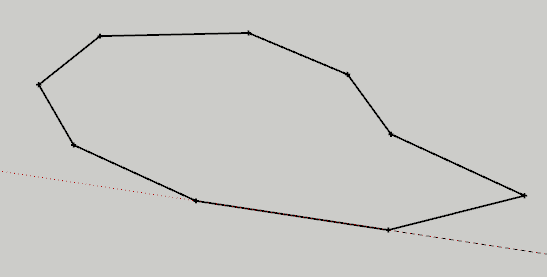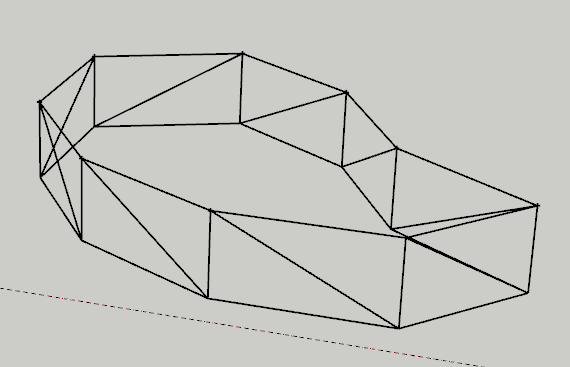# Generating triangles with normals from line strip

Hello

I have a home-made program for my luthery activity as a hobbyist that computes a BMP with contour lines from another BMP with the outside contour line of the arch, the center line and the arch height.
It perfectly works.

Now I’d like this program to generate 2 3D models (front and back plates) from these computed values.
I have made the code to generate a 2D list of the outside points separated from a constant distance of the instrument (that I could draw as a line strip, so). I would like not to produce 4 times the vertices to draw the side of the plates.
So here is what I have:and here is what I want to display as the side of the plates:I’m new to geometry shaders but I quite see how to generate the triangles from these line strips. Now, talking about normals, my code calculates 2D normals with each vertex according the position of the neighbour ones. I would like the final triangles to have the correct normals for each generated vertex. The calculation is easy:
For the top vertex, Nx*=sqrt(2); Ny=sqrt(2); Nz*=sqrt(2);
For the top vertex, Nx*=sqrt(2); Ny=-sqrt(2); Nz*=sqrt(2);
I would like to know how to programmatically set the normals for these computed 3D vertices from the 2D points normals passed to the shaders, please? I haven’t seen any example of a normal-generating GS.

I hope I am intelligible enough

Thanks for the help
Cathy L.